$$\def\RR{\bf R} \def\real{\mathbb{R}} \def\bold#1{\bf #1} \def\d{\mbox{Cord}} \def\hd{\widehat \mbox{Cord}} \DeclareMathOperator{\cov}{cov} \DeclareMathOperator{\var}{var} \DeclareMathOperator{\cor}{cor} \newcommand{\ac}{\left\{#1\right\}} \DeclareMathOperator{\Ex}{\mathbb{E}} \DeclareMathOperator{\diag}{diag}$$

## From Big Data to Knowledge: Brain, Twitter, and Beyond

### Xi (Rossi) Luo

Brown University
Department of Biostatistics
Center for Statistical Sciences
Computation in Brain and Mind
Brown Institute for Brain Science
Brown Data Science InitiativeApril 19, 2017

Slides at bit.ly/data1704

## Big Data and Brain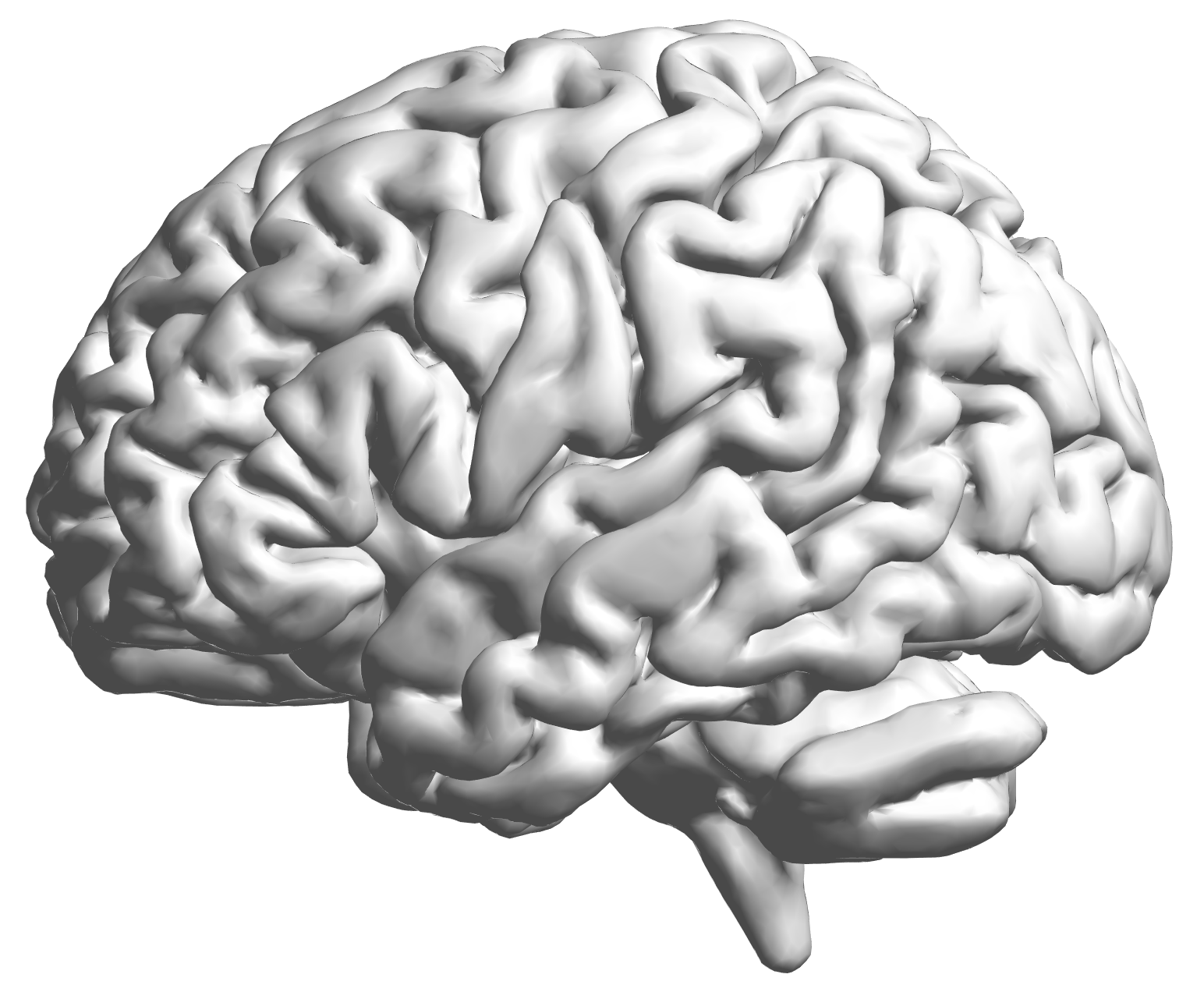• 100 million ( $10^{11}$) neurons
• 100 trillion ( $10^{14}$) neuronal connectionsfMRI data: blood-oxygen-level dependent (BOLD) signals from each cube/voxel (~millimeters), $10^5$ ~ $10^6$ voxels in total.

## Complex Data: fMRI Studies

Sub 1, Sess 1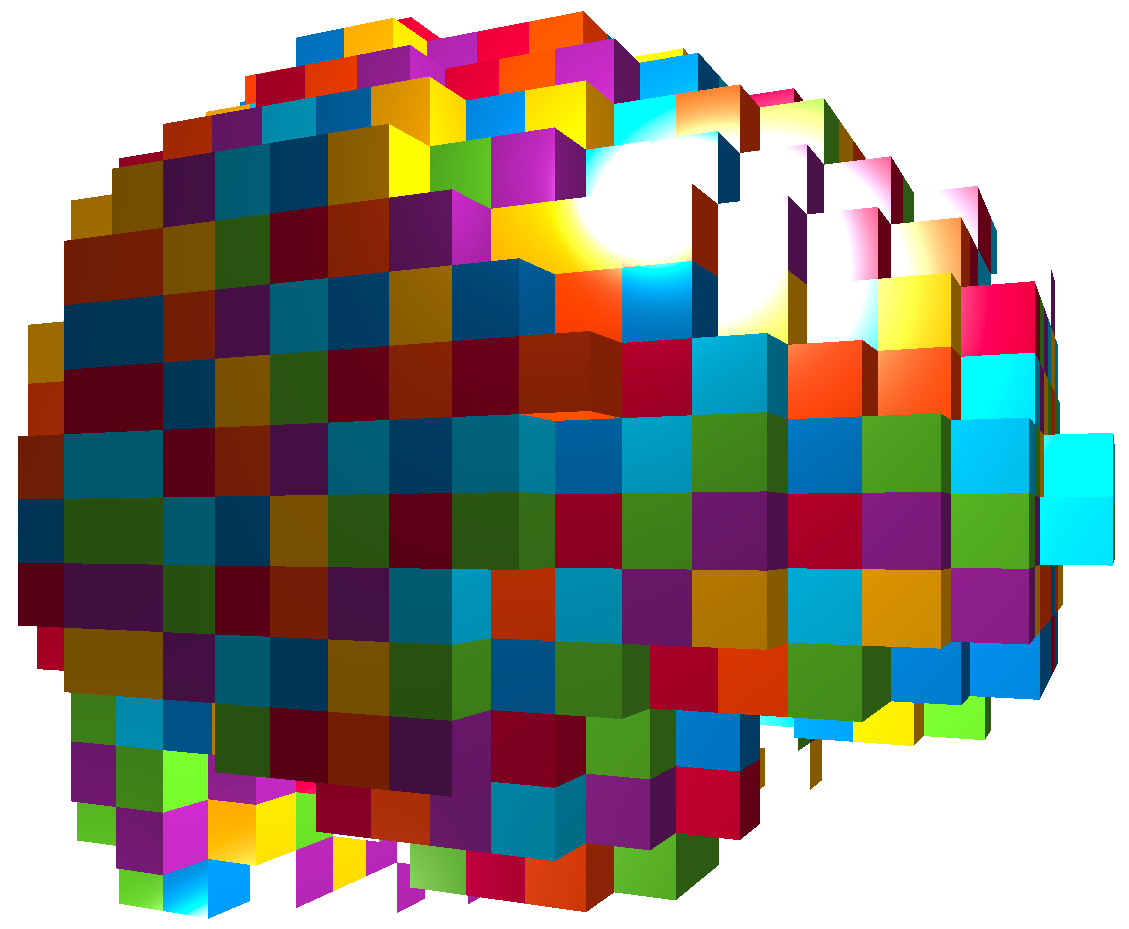Time 12~200

Sub i, Sess jSub ~100, Sess ~4Large, multilevel (subject, sess, voxel) data
$100 \times 4 \times 300 \times 10^6 \approx 100$ billion data points

## Predicting Cocaine Relapses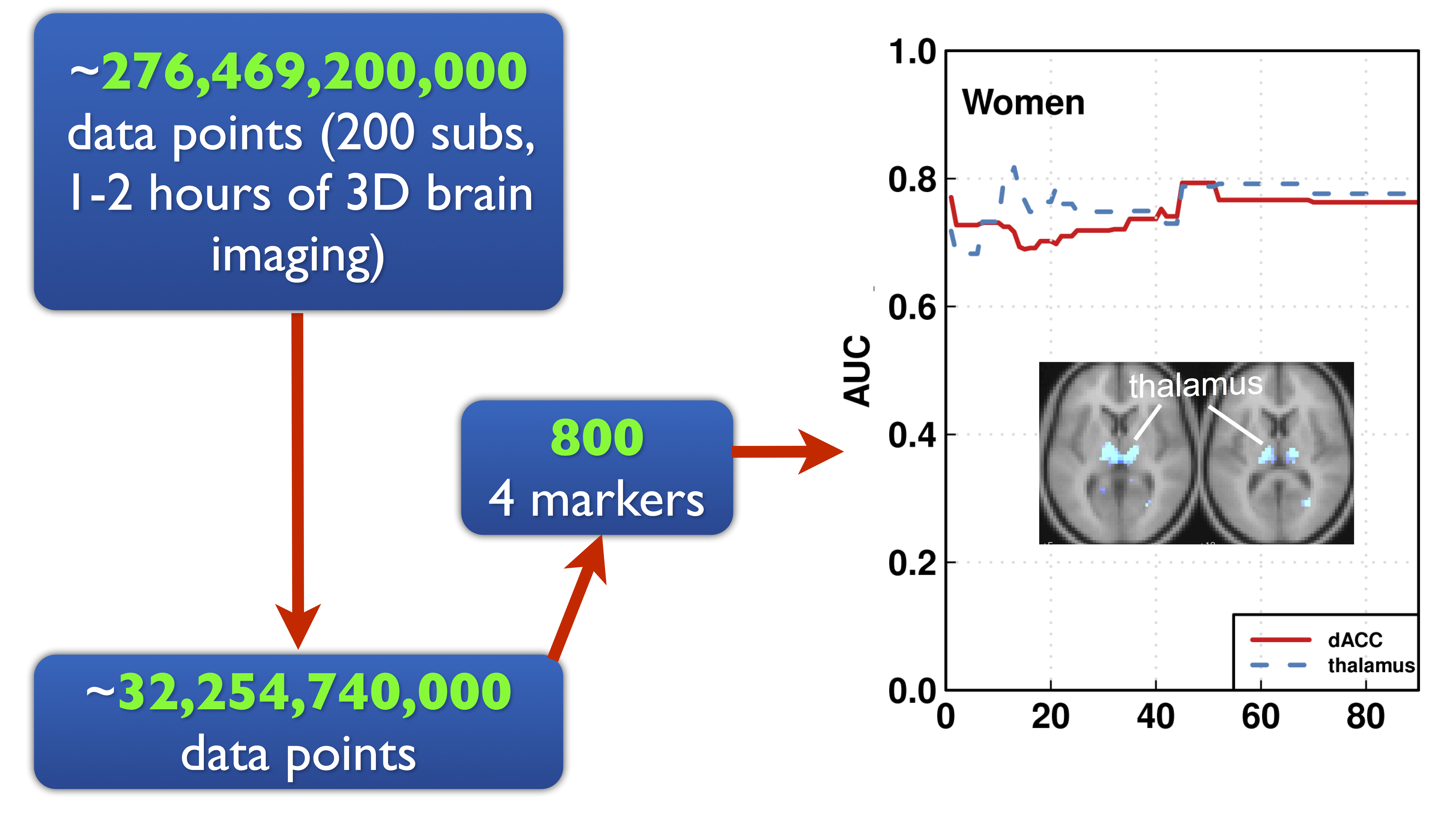Image: Luo et al, 13

## Complex Models: Brain Networks

Find brain networks with 1 trillion ( $10^{12}$) possible connections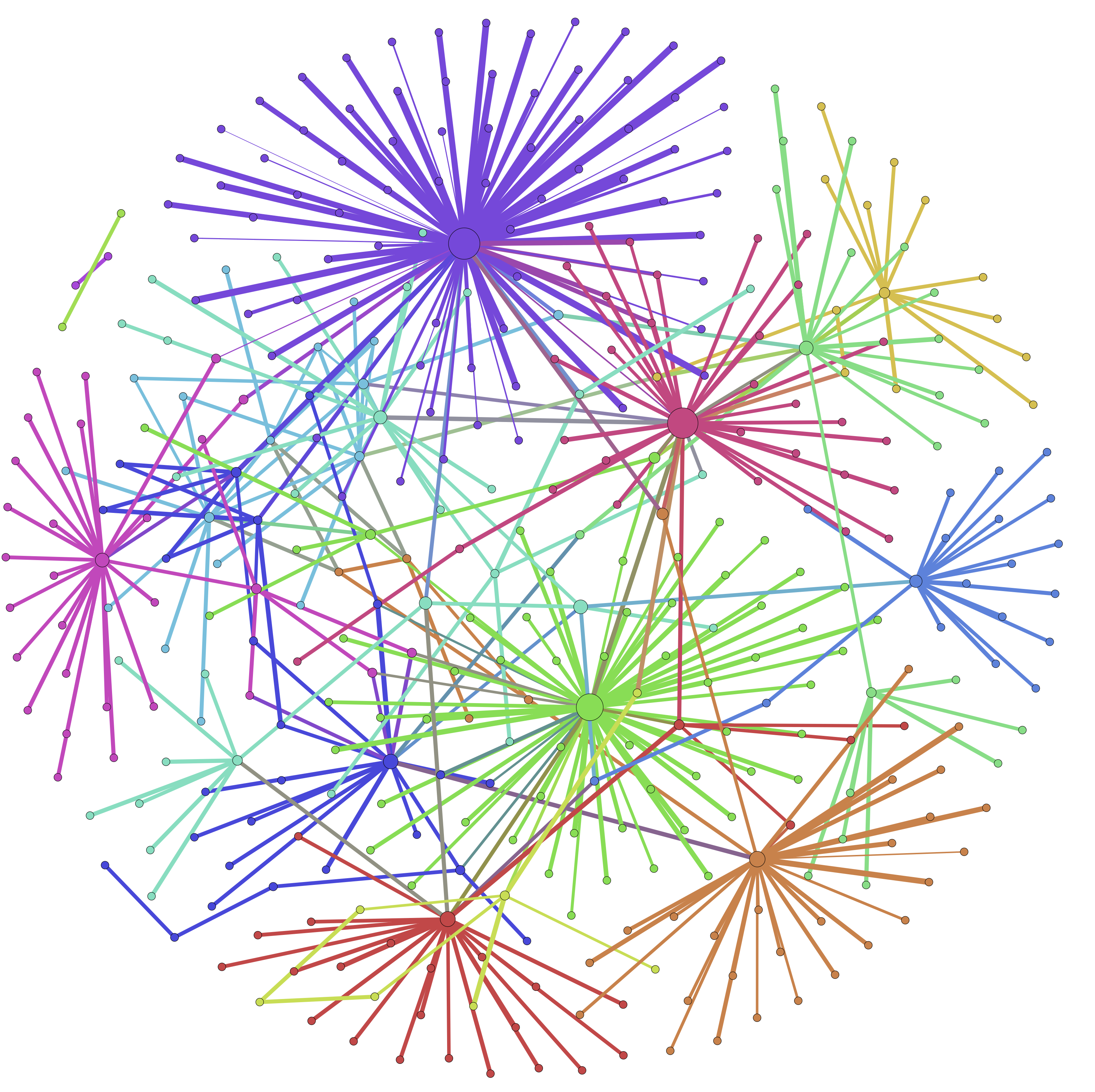Image: Liu and Luo, 15

## Course: Stat Learning and Big Data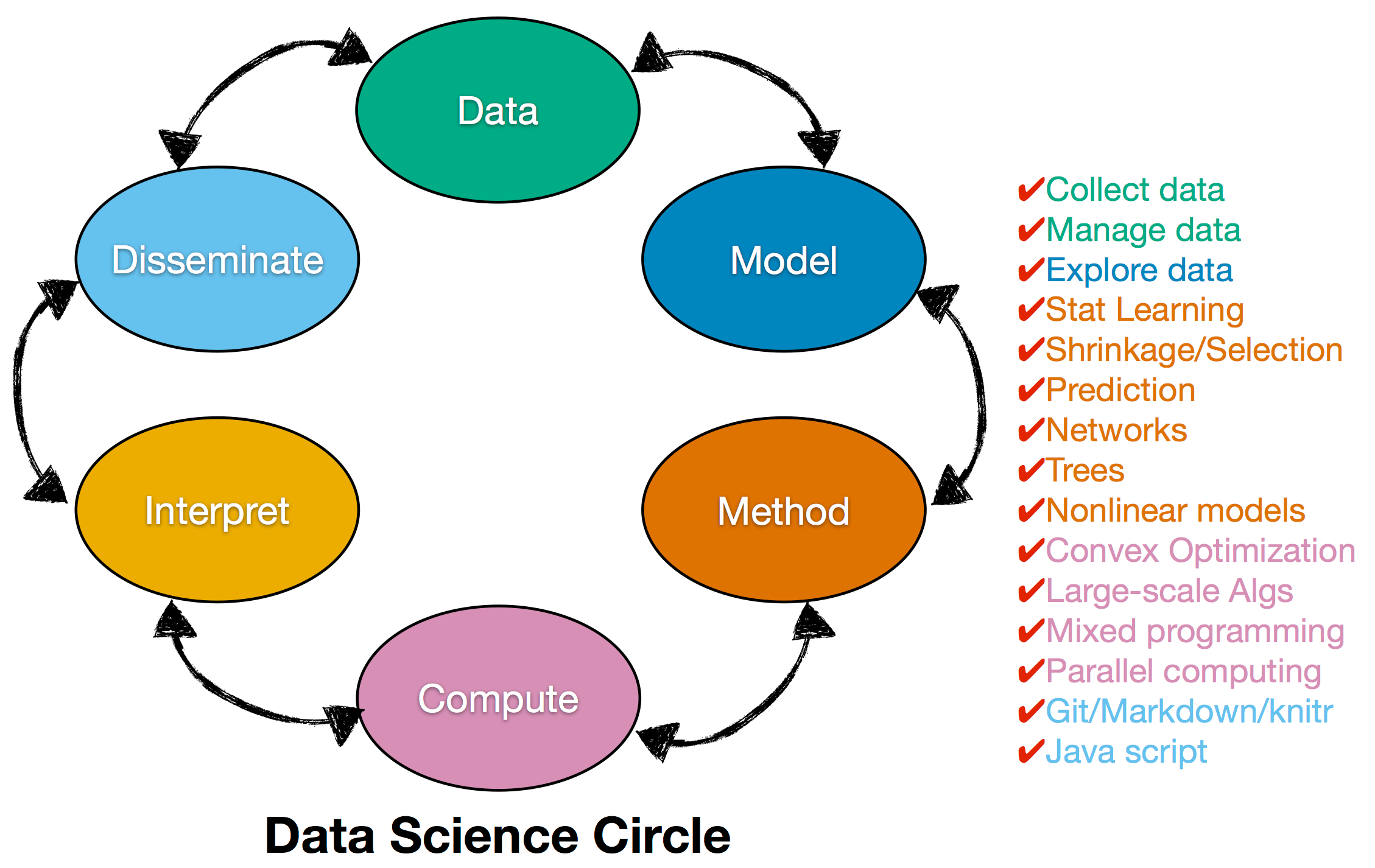Applied Mathematics + Computer Science + Statistics

Thanks to the support of Office of the Provost, the Sheridan Center, and School of Professional Studeis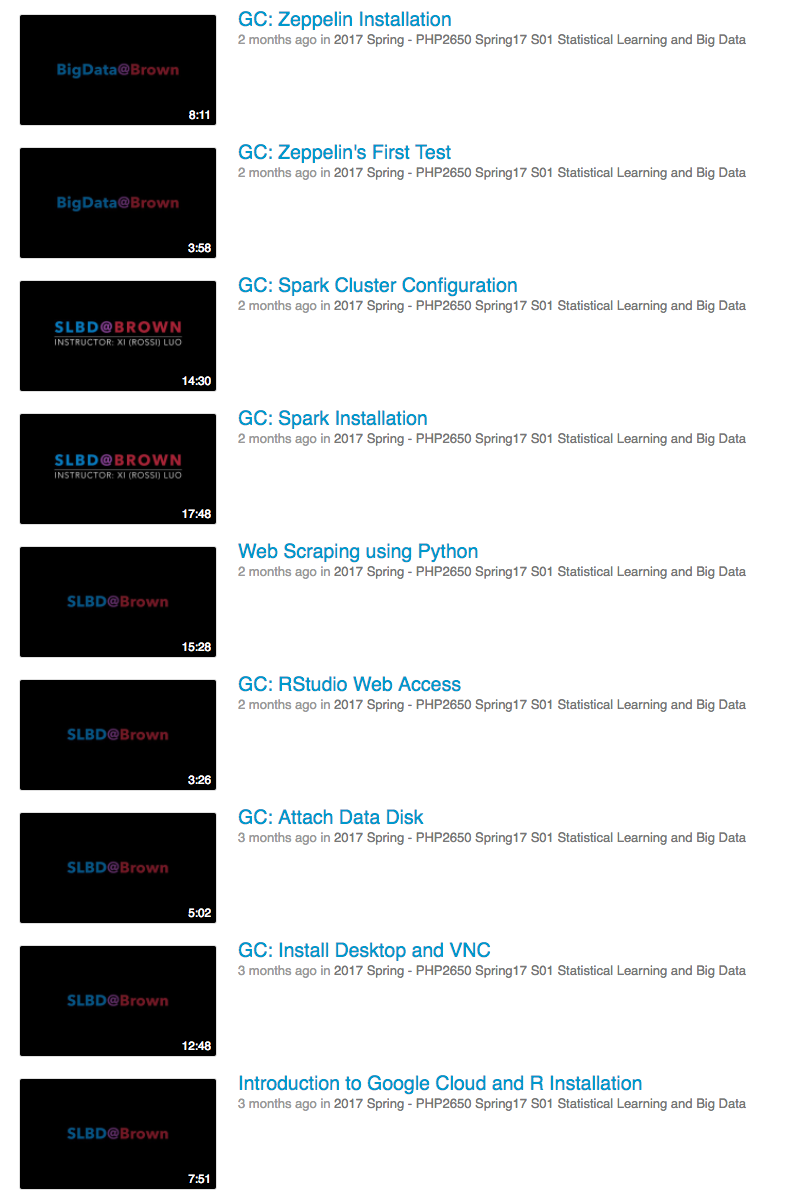Video tutorials on cloud computing, Hadoop, and many other big data technologiesHillary ClintonBernie Sanders

Imge: wikipedia

## Excerpt of DataJaime Zhang, student in SMBDStatistical test: $p \lt 10^{-4}$

Jaime Zhang, student in SMBD## Summary

• Big data extraction and management
• Complex mathematical modeling
• Extract knowledge from data
• Course PHP 2650 SLBD covers the above points